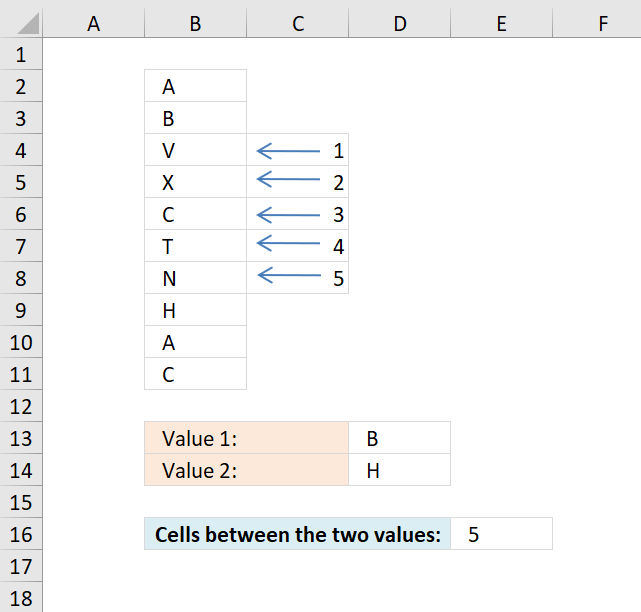Author: Oscar Cronquist Article last updated on December 17, 2018The formula in cell E16 counts the number of cells between value B and H, Value B is in cell B3 and H is in B9, cells B4, B5, B6, B7 and B8 are in between.

=ABS(MATCH(D13, B2:B11, 0)-MATCH(D14, B2:B11, 0))-1

### Explaining formula in cell E16

#### Step 1 - Find value in cell D13 in cell range B2:B11

The MATCH function finds the relative position of a value in an array or cell range.

MATCH(D13, B2:B11, 0)

becomes

MATCH("B", {"A"; "B"; "V"; "X"; "C"; "T"; "N"; "H"; "A"; "C"}, 0)

and returns 2.

#### Step 2 - Find value in cell D14 in cell range B2:B11

MATCH(D14, B2:B11, 0)

becomes

MATCH("H", {"A"; "B"; "V"; "X"; "C"; "T"; "N"; "H"; "A"; "C"}, 0)

and returns 8.

#### Step 3 - Subtract positions

MATCH(D13, B2:B11, 0)-MATCH(D14, B2:B11, 0)

becomes

2-8 equals -6.

#### Step 4 - Remove sign

We don't know where the values are in the cell range so it may happen that we get a negative number from time to time, this example is such occasion. The ABS function removes the sign from a number.

ABS(MATCH(D13, B2:B11, 0)-MATCH(D14, B2:B11, 0))

becomes

ABS(-6)

and returns 6.

#### Step 5 - Subtract with 1

The calculation counts the last cell as well, we only need the cells in between.

ABS(MATCH(D13, B2:B11, 0)-MATCH(D14, B2:B11, 0))-1

becomes

6-1

and returns 5 in cell E16.

### Get Excel *.xlsx file

formula to count cells between two values.xlsx